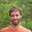Question-and-Answer Resource for the Building Energy Modeling Community
Get started with the Help page

Numerical equations with EMS

Hello,

I am trying to write a subroutine that calculates the temperature of my storage medium at each timestep. I am trying to apply a finite difference model that solves N ODEs for each node, the model is very similar to that of WaterHeater:Stratified where the temperature update equation for each node is:

Tfinal= (Tinitial +b/a).exp(a.deltaT)-b/a

Does EMS allow this sort of formulations ?

My idea is to create a trend variable for the temperature at each node, but I don`t understand how initialization works in EMS Any insights? Thank you

edit retag close merge delete

Sort by » oldest newest most votedIf I'm understanding correctly, you do not need to use a trend variable just to retrieve a variable's value from a previous timestep. Rather, just use an EnergyManagementSystem:GlobalVariable. For example:

EnergyManagementSystem:Program,
TemperatureCalculation,
SET Tfinal = Tinitial + 10,
SET Tinitial = Tfinal;

And then set Tinitial as a global EMS variable so that its value at the end of the previous timestep can be used in the program in the subsequent timestep.

If you need to set the initial value for Tinitial for the beginning of the simulation, you can do this by creating a second EMS program that sets it value (e.g., SET Tinitial = 50) and uses the BeginNewEnvironment and/or AfterNewEnvironmentWarmUpIsComplete calling points (I forget which offhand).

more

Thank you very much this is really helpful!